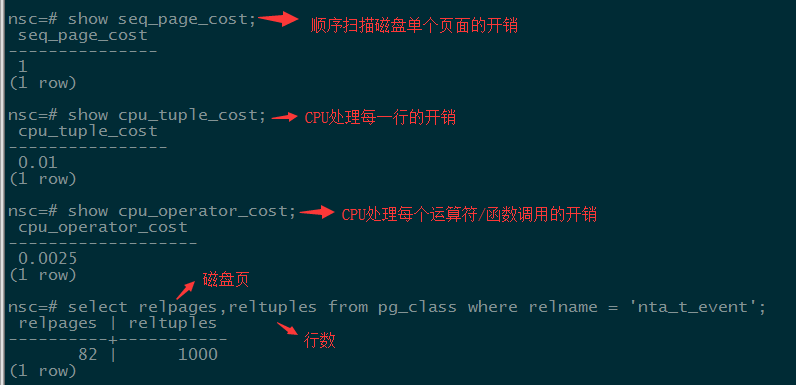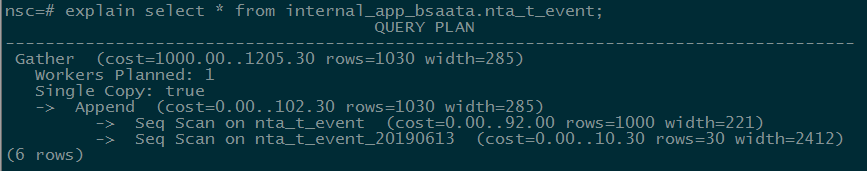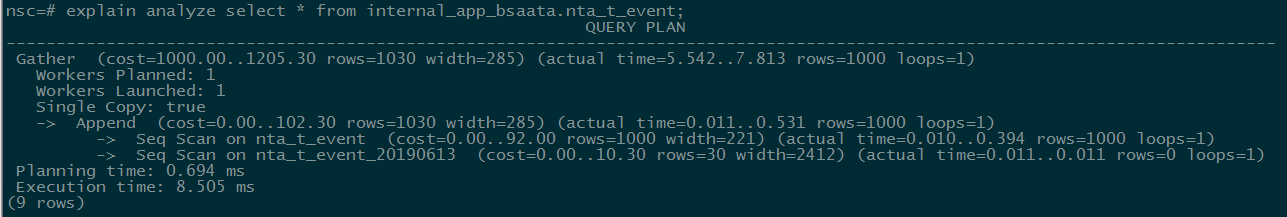PostgreSQL是“世界上最先进的开源关系型数据库”。因为出现较晚，所以客户人群基数较MySQL少，但是发展势头很猛，最大优势是完全开源。

pg在查询规划路径过程中，查询请求的不同执行方案是通过建立不同的路径来表达的，在生成较多符合条件的路径之后，要从中选择出代价最小的路径，把它转化为一个执行计划，传递给执行器执行。那么如何生成最小代价的计划呢？基于统计信息估计计划中各个节点的成本，其中与之相关的参数如下所示：``````# 估算代价：
total_cost = seq_page_cost * relpages + cpu_tuple_cost *  reltuples

# 有时我们不想用系统默认的执行计划，这时可以通过禁止/开启某种运算的语法来强制控制执行计划：
enable_bitmapscan = on
enable_hashagg = on
enable_hashjoin = on
enable_indexscan = on #索引扫描
enable_indexonlyscan = on #只读索引扫描
enable_material = on #物化视图
enable_mergejoin = on
enable_nestloop = on
enable_seqscan = on
enable_sort = on
enable_tidscan = on

# 按照上面扫描方式并过滤代价：
Cost = seq_page_cost * relpages + cpu_tuple_cost *  reltuples + cpu_operation_cost * reltuples
``````

``````nsc=# \h explain;
Command:     EXPLAIN
Description: show the execution plan of a statement
Syntax:
EXPLAIN [ ( option [, ...] ) ] statement
EXPLAIN [ ANALYZE ] [ VERBOSE ] statement

where option can be one of:

ANALYZE [ boolean ]  -- 是否真正执行，默认false
VERBOSE [ boolean ]  -- 是否显示详细信息，默认false
COSTS [ boolean ]    -- 是否显示代价信息，默认true
BUFFERS [ boolean ]  -- 是否显示缓存信息，默认false，前置事件是analyze
TIMING [ boolean ]   -- 是否显示时间信息
FORMAT { TEXT | XML | JSON | YAML }  -- 输格式，默认为text
``````Seq Scan（全表扫描）：把表的所有数据块从头到尾读一遍，筛选出符合条件的数据块；

Index Scan（索引扫描）：为了加快查询速度，在索引中找到需要的数据行的物理位置，再到表数据块中把对应数据读出来，如B树，GiST，GIN，BRIN，HASH

Bitmap Index/Heap Scan（位图索引/结果扫描）：把满足条件的行或块在内存中建一个位图，扫描完索引后，再根据位图列表的数据文件把对应的数据读出来，先通过Bitmap Index Scan在索引中找到符合条件的行，在内存中建立位图，之后再到表中扫描Bitmap Heap Scan。

Materialize：对下层节点返回的元组进行缓存（如连接表时）

Sort：对下层返回的节点进行排序（如果内存超过iwork_mem参数指定大小，则节点工作空间切换到临时文件，性能急剧下降）

Group：对下层排序元组进行分组操作

Agg：执行聚集函数（sum/max/min/avg）

Nestedloop Join（嵌套连接）： 内表被外表驱动，外表返回的每一行都要在内表中检索找到与它匹配的行，因此整个查询返回的结果集不能太大，要把返回子集较小的表作为外表，且内表的连接字段上要有索引。 执行过程为，确定一个驱动表（outer table），另一个表为inner table，驱动表中每一行与inner table中的相应记录关联；

Hash Join（哈希连接）：优化器使用两个比较的表，并利用连接属性在内存中建立散列表，然后扫描较大的表并探测散列表，找出与散列表匹配的行；

Merge Join（合并连接）：通常hash连接的性能要比merge连接好，但如果源数据上有索引，或结果已经被排过序，这时merge连接性能会优于hash连接；

Seq Scan顺序扫描表无启动时间
Index Scan索引扫描无启动时间
Bitmap Index Scan索引扫描有启动时间
Bitmap Heap Scan索引扫描有启动时间
Subquery Scan子查询无启动时间
Tid Scan行号检索无启动时间
Function Scan函数扫描无启动时间
Nested Loop Join嵌套连接无启动时间
Merge Join合并连接有启动时间
Hash Join哈希连接有启动时间
Sort排序(order by)有启动时间
Hash哈希运算有启动时间
Result函数扫描，和具体的表无关无启动时间
Uniquedistinct/union有启动时间
Limitlimit/offset有启动时间
Aggregatecount, sum,avg等聚集函数有启动时间
Groupgroup by有启动时间
Appendunion操作无启动时间
Materialize子查询有启动时间
SetOpintersect/except有启动时间

``````SELECT
te.event_type,
sum(tett.feat_bytes) AS traffic
FROM t_event te
LEFT JOIN t_event_traffic_total tett
ON tett.event_id = te.event_id
WHERE
((te.event_type >= 1 AND te.event_type <= 17) OR (te.event_type >= 23 AND te.event_type <= 26) OR (te.event_type >= 129 AND te.event_type <= 256))
AND te.end_time >= '2017-10-01 09:39:41+08:00'
AND te.begin_time <= '2018-01-01 09:39:41+08:00'
AND tett.stat_time >= '2017-10-01 09:39:41+08:00'
AND tett.stat_time < '2018-01-01 09:39:41+08:00'
GROUP BY te.event_type
ORDER BY total_count DESC
LIMIT 10
``````

``````select count(1) from t_event;  -- 535881条
select count(1) from t_event_traffic_total; -- 2123235条
``````

``````event_type    traffic
17	2.26441505638877E17
2	2.25307250128674E17
7	1.20629298837E15
26	285103860959500
1	169208970599500
13	47640495350000
6	15576058500000
3	12671721671000
15	1351423772000
11	699609230000
``````

``````Limit  (cost=5723930.01..5723930.04 rows=10 width=12) (actual time=3762.383..3762.384 rows=10 loops=1)
Output: te.event_type, (sum(tett.feat_bytes))
->  Sort  (cost=5723930.01..5723930.51 rows=200 width=12) (actual time=3762.382..3762.382 rows=10 loops=1)
Output: te.event_type, (sum(tett.feat_bytes))
Sort Key: (sum(tett.feat_bytes))
Sort Method: quicksort  Memory: 25kB
->  HashAggregate  (cost=5723923.69..5723925.69 rows=200 width=12) (actual time=3762.360..3762.363 rows=18 loops=1)
Output: te.event_type, sum(tett.feat_bytes)
->  Merge Join  (cost=384982.63..4390546.88 rows=266675361 width=12) (actual time=2310.395..3119.886 rows=2031023 loops=1)
Output: te.event_type, tett.feat_bytes
Merge Cond: (te.event_id = tett.event_id)
->  Sort  (cost=3284.60..3347.40 rows=25119 width=12) (actual time=21.509..27.978 rows=26225 loops=1)
Output: te.event_type, te.event_id
Sort Key: te.event_id
Sort Method: external merge  Disk: 664kB
Buffers: shared hit=652, temp read=84 written=84
->  Append  (cost=0.00..1448.84 rows=25119 width=12) (actual time=0.027..7.975 rows=26225 loops=1)
Buffers: shared hit=652
->  Seq Scan on public.t_event te  (cost=0.00..0.00 rows=1 width=12) (actual time=0.001..0.001 rows=0 loops=1)
Output: te.event_type, te.event_id
Filter: ((te.end_time >= '2017-10-01 09:39:41+08'::timestamp with time zone) AND (te.begin_time <= '2018-01-01 09:39:41+08'::timestamp with time zone) AND (((te.event_type >= 1) AND (te.event_type <= 17)) OR ((te.event_type >= 23) AND (te.event_type <= 26)) OR ((te.event_type >= 129) AND (te.event_type <= 256))))
->  扫描子表过程，省略...
->  Materialize  (cost=381698.04..392314.52 rows=2123296 width=16) (actual time=2288.881..2858.256 rows=2123235 loops=1)
Output: tett.feat_bytes, tett.event_id
->  Sort  (cost=381698.04..387006.28 rows=2123296 width=16) (actual time=2288.877..2720.994 rows=2123235 loops=1)
Output: tett.feat_bytes, tett.event_id
Sort Key: tett.event_id
Sort Method: external merge  Disk: 53952kB
->  Append  (cost=0.00..49698.20 rows=2123296 width=16) (actual time=0.026..470.610 rows=2123235 loops=1)
->  Seq Scan on public.t_event_traffic_total tett  (cost=0.00..0.00 rows=1 width=16) (actual time=0.001..0.001 rows=0 loops=1)
Output: tett.feat_bytes, tett.event_id
Filter: ((tett.stat_time >= '2017-10-01 09:39:41+08'::timestamp with time zone) AND (tett.stat_time < '2018-01-01 09:39:41+08'::timestamp with time zone))
->  扫描子表过程，省略...
Total runtime: 3771.346 ms
``````